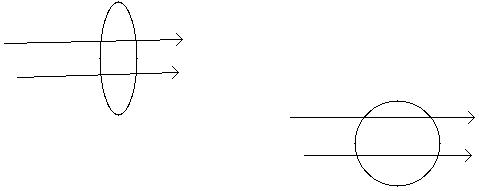Question# To monitor the breathing of a hospital patient, a thin belt isgirded around the patient's chest. The belt is a200-turn coil. When the patient inhales,

Other
ANSWEREDTo monitor the breathing of a hospital patient, a thin belt isgirded around the patient's chest. The belt is a200-turn coil. When the patient inhales, the area encircled by the coil increases by $$\displaystyle{39.0}{c}{m}^{{2}}$$. The magnitude of the Earth's magnetic field is 50.0uT and makes an angle of 28.0 degree with the plane of the coil. Assuming a patien takes 1.80s toin hale, find the magnitude of the average induced emf in the coilduring that time.
Do I use the equation $$\displaystyle{E}={N}\cdot{A}\cdot{B}{w}{\sin{{w}}}{t}$$?2021-04-25
You were close. This is Faraday's Law of Induction.
The flux is $$\displaystyle\phi={N}{B}{A}{\cos{\theta}}$$
N is the number of turns
B is the strength of the magnetic field (should be in T)
A is the area of the loop (should be in $$\displaystyle{m}^{{2}}$$)
The angle is between the magnetic field and the normal(perpendicular to the coil) so it would be cos(90.0 - 28.0).
The EMF = - rate of change in flux, or
$$\displaystyle{E}{M}{F}={\frac{{-\triangle\phi}}{{\triangle{t}}}}$$
The $$\displaystyle\triangle\phi={N}{B}{\left(\triangle{A}\right)}{\cos{\theta}}$$ (since A is the only thingthat changes, and you don't need the original A, only the change in A)
$$\displaystyle\triangle={1.80}{s}$$
If the above still confuses you...
Flux Linkage, or simply flux, refers to the magnetic field linespassing through the area of a loop:The diagram on the left shows the loop allows the magnetic fieldvectors to pass through it ($$\displaystyle\theta$$ ˜ 0 deg), but on the right,the magnetic field vectors cannot pass through it ($$\displaystyle\theta$$ = 90deg).
The flux is $$\displaystyle\phi={N}{B}{A}{\cos{\theta}}$$, so in the case on the cos (0deg) = 1, so $$\displaystyle\phi={N}{B}{A}$$, but on the right cos (90 deg) = 0, so $$\displaystyle\phi={0}$$.
The way that $$\displaystyle\theta$$ is defined is it is perpendicular to the planeof the loops. Think about how the diagram on the right has a $$\displaystyle\theta$$= 90 deg. The area vector is pointing straight out at us in theright figure.